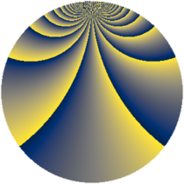# Properties

 Label 900.3.buLevel $900$ Weight $3$ Character orbit 900.bu Rep. character $\chi_{900}(23,\cdot)$ Character field $\Q(\zeta_{60})$ Dimension $5696$ Sturm bound $540$

# Related objects

## Defining parameters

 Level: $$N$$ $$=$$ $$900 = 2^{2} \cdot 3^{2} \cdot 5^{2}$$ Weight: $$k$$ $$=$$ $$3$$ Character orbit: $$[\chi]$$ $$=$$ 900.bu (of order $$60$$ and degree $$16$$) Character conductor: $$\operatorname{cond}(\chi)$$ $$=$$ $$900$$ Character field: $$\Q(\zeta_{60})$$ Sturm bound: $$540$$

## Dimensions

The following table gives the dimensions of various subspaces of $$M_{3}(900, [\chi])$$.

Total New Old
Modular forms 5824 5824 0
Cusp forms 5696 5696 0
Eisenstein series 128 128 0

## Trace form

 $$5696q - 24q^{2} - 10q^{4} - 48q^{5} - 12q^{6} - 40q^{9} + O(q^{10})$$ $$5696q - 24q^{2} - 10q^{4} - 48q^{5} - 12q^{6} - 40q^{9} - 32q^{10} - 34q^{12} - 16q^{13} - 30q^{14} - 6q^{16} + 4q^{18} - 24q^{20} - 24q^{21} - 24q^{22} - 16q^{25} - 96q^{28} - 60q^{29} + 54q^{30} + 156q^{32} - 68q^{33} - 10q^{34} + 52q^{36} - 64q^{37} - 8q^{40} - 36q^{41} + 1202q^{42} - 132q^{45} - 24q^{46} + 52q^{48} - 24q^{50} + 56q^{52} + 630q^{54} - 18q^{56} + 8q^{57} - 24q^{58} - 1838q^{60} - 12q^{61} - 40q^{64} + 240q^{65} - 156q^{66} + 72q^{68} - 40q^{69} - 108q^{70} - 230q^{72} - 64q^{73} + 48q^{76} - 48q^{77} + 310q^{78} - 24q^{81} - 16q^{82} - 20q^{84} - 16q^{85} - 18q^{86} + 96q^{88} - 86q^{90} - 366q^{92} - 668q^{93} + 590q^{94} - 12q^{96} - 16q^{97} + O(q^{100})$$

## Decomposition of $$S_{3}^{\mathrm{new}}(900, [\chi])$$ into newform subspaces

The newforms in this space have not yet been added to the LMFDB.